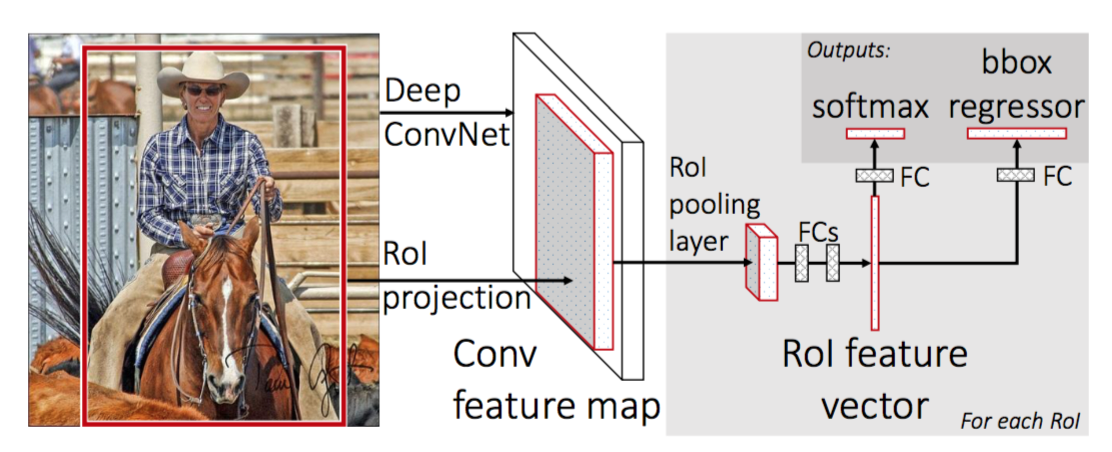# Fast R-CNNPhoto by jet dela cruz on Unsplash

# Overview

• Apply fully Convolutional networks to the whole image.
• ROI Pooling: each proposal is pooled into a fixed-size feature map.
• Classification with a softmax layer.
• Regression-based bounding box refinement.

# Architecture

A Fast R-CNN network takes as input an entire image and a set of object proposals.
1. The network first processes the whole image with several convolutional (conv) and max-pooling layers to produce a conv feature map.
2. For each object proposal a region of interest (RoI) pooling layer extracts a fixed-length feature vector from the feature map.
3. Each feature vector is fed into a sequence of fully connected (FC) layers that finally branch into two sibling output layers:

• One that produces softmax probability estimates over K object classes plus a catch-all “background” class.
• Another layer that outputs four real-valued numbers for each of the K object classes (Each set of 4 values encodes refined bounding-box positions for one of the K classes).Fast R-CNN architecture image in the paper

## ROI pooling

The RoI pooling layer uses max pooling to convert the features inside any valid region of interest into a small feature map with a fixed spatial extent of H and W (e.g., 7 7), where H and W are layer hyper-parameters that are independent of any particular RoI.

How it works :

1. Dividing the region proposal into equal-sized sections (the number of which is the same as the dimension of the output)
2. Finding the largest value in each section
3. Copying these max values to the output buffer

## Multi task loss

A Fast R-CNN network has two sibling output layers.

Note: The case where u=0 is ignored because the background classes have no-+ ground truth boxes.

• The first output is a discrete probability distribution (per RoI), p = (p0,…,pK), over K + 1 categories.As usual, p is computed by a softmax over the K+1 outputs of a fully connected layer:
• The second sibling layer produces 4 bounding box regression offsets tᵏᵢ where i = x, y, w, and h. (x, y) stands for the top-left corner and w and h denote the height and width of the bounding box. The true bounding box regression targets for a class u are indicated by vᵢ where i = x, y, w, and h when u≥1:

Multi-task training is convenient because it avoids managing a pipeline of sequentially-trained tasks. But it also has the potential to improve results because the tasks influence each other through a shared representation.

## Truncated SVD

The large fully connected layers can be compressed with truncated SVD to make the network more efficient. Here a layer parameterized by W as its weight matrix can be factorized to reduce the parameter count by splitting it into two layers (ΣₜVᵀ and U with biases) without a non-linearity between them, where W ~ U ΣₜVᵀ.

# Some Details in Paper

• At runtime, the detection network processes images in 0.3s.
• We experiment with three pre-trained ImageNet networks each with five max-pooling layers and between five and thirteen conv layers.
• Training all network weights with back-propagation is an important capability of Fast R-CNN.
• In Fast RCNN training, stochastic gradient descent (SGD) mini-batches are sampled hierarchically, first by sampling N images and then by sampling R/N RoIs from each image.
• During training, each mini-batch is constructed from N=2 images. The mini-batch consists of 64 ROIs from each image.
• During training, images are horizontally flipped with a probability of 0.5. No other data augmentation is used.
• Like R-CNN, 25% of the ROIs are object proposals that have at least 0.5 IoU with a ground-truth bounding box of a foreground class. These would be positive for that particular class and would be labeled with the appropriate u=1…K. The remaining ROIs are sampled from proposals that have an IoU with ground-truth bounding boxes between [0.1, 0.5). These ROIs are labeled as belonging to the class u = 0 (background class).
• The fully connected layers used for softmax classification and bounding-box regression are initialized from zero-mean Gaussian distributions with standard deviations 0.01 and 0.001, respectively. Biases are initialized to 0.
• In the multi-scale pipeline of Fast R-CNN, input images are resized into a randomly sampled size at train time to introduce scale invariance. At test time, each image is fed to the network in multiple fixed scales. For each ROI, the features are pooled from only one of these scales, chosen such that the scaled candidate window has the number of pixels closest to 224 x 224. However, the authors find that the single-scale pipeline performs almost as well with a much lower computing time cost. In the single-scale approach, all images at train and test time are resized to 600 (shortest side) with the upper cap on the longest side being 1000.

# Advantages of Fast R-CNN over R-CNN and SPP

1. Higher detection quality (mAP) than R-CNN, SPPnet

2. Training is single-stage, using a multi-task loss

3. Training can update all network layers

4. No disk storage is required for feature caching

# Problems with Fast R-CNN

Region generator (selective search) is a fixed algorithm so it doesn’t optimize during training.

References

 Girshick, Ross et al. “Rich Feature Hierarchies for Accurate Object Detection and Semantic Segmentation.” 2014 IEEE Conference on Computer Vision and Pattern Recognition (2014)
 Girshick, Ross. “Fast R-CNN.” 2015 IEEE International Conference on Computer Vision (ICCV) (2015)
 He, Kaiming et al. “Spatial Pyramid Pooling in Deep Convolutional Networks for Visual Recognition.” Lecture Notes in Computer Science (2014)
 Uijlings, J. R. R. et al. “Selective Search for Object Recognition.” International Journal of Computer Vision 104.2 (2013)

--

--